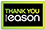# Introduction to computational mathematics

Category: Mathematics
"This book is excellent and can be recommended for undergraduates and graduates to acquire rapidly the basic knowledge in computational mathematics." This unique book provides a comprehensive introduction to computational mathematics, which forms an essential part of contemporary numerical algorithms, scientific computing and optimization. It uses a theorem-free approach with just the right balance between mathematics and numerical algorithms. This edition covers all major topics in computational mathematics with a wide range of carefully selected numerical algorithms, ranging from the root-finding algorithm, numerical integration, numerical methods of partial differential equations, finite element methods, optimization algorithms, stochastic models, nonlinear curve-fitting to data modelling, bio-inspired algorithms and swarm intelligence. This book is especially suitable for both undergraduates and graduates in computational mathematics, numerical algorithms, scientific computing, mathematical programming, artificial intelligence and engineering optimization. Thus, it can be used as a textbook and/or reference book.
€13.6841 Reward Points
Currently out of stock
Orders will not be processed until after the current Coronavirus (COVID-19) restrictions are lifted

Any purchases for more than €10 are eligible for free delivery anywhere in the UK or Ireland!

"This book is excellent and can be recommended for undergraduates and graduates to acquire rapidly the basic knowledge in computational mathematics." This unique book provides a comprehensive introduction to computational mathematics, which forms an essential part of contemporary numerical algorithms, scientific computing and optimization. It uses a theorem-free approach with just the right balance between mathematics and numerical algorithms. This edition covers all major topics in computational mathematics with a wide range of carefully selected numerical algorithms, ranging from the root-finding algorithm, numerical integration, numerical methods of partial differential equations, finite element methods, optimization algorithms, stochastic models, nonlinear curve-fitting to data modelling, bio-inspired algorithms and swarm intelligence. This book is especially suitable for both undergraduates and graduates in computational mathematics, numerical algorithms, scientific computing, mathematical programming, artificial intelligence and engineering optimization. Thus, it can be used as a textbook and/or reference book.
Currently out of stock
Orders will not be processed until after the current Coronavirus (COVID-19) restrictions are lifted41 Reward Points

Any purchases for more than €10 are eligible for free delivery anywhere in the UK or Ireland!

€13.68
Currently out of stock
Orders will not be processed until after the current Coronavirus (COVID-19) restrictions are lifted41 Reward Points

Any purchases for more than €10 are eligible for free delivery anywhere in the UK or Ireland!

### Product Description

"This book is excellent and can be recommended for undergraduates and graduates to acquire rapidly the basic knowledge in computational mathematics." This unique book provides a comprehensive introduction to computational mathematics, which forms an essential part of contemporary numerical algorithms, scientific computing and optimization. It uses a theorem-free approach with just the right balance between mathematics and numerical algorithms. This edition covers all major topics in computational mathematics with a wide range of carefully selected numerical algorithms, ranging from the root-finding algorithm, numerical integration, numerical methods of partial differential equations, finite element methods, optimization algorithms, stochastic models, nonlinear curve-fitting to data modelling, bio-inspired algorithms and swarm intelligence. This book is especially suitable for both undergraduates and graduates in computational mathematics, numerical algorithms, scientific computing, mathematical programming, artificial intelligence and engineering optimization. Thus, it can be used as a textbook and/or reference book.

### Product Details

#### Introduction to computational mathematics

ISBN9789814635783

Format

PublisherWSPC (26 January. 2015)

No. of Pages0

Weight498

Language English (United States)

Dimensions 230 x 153 x 23